Next: 2.1 Graphene Up: Dissertation Hossein Karamitaheri Previous: 1.5 Graphene- and Silicon-based Nanostructures   Contents

# 2. Physical Models

The thermoelectric figure of merit for materials is defined as: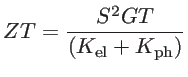(2.1)

where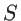is the Seebeck coefficient,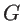the electrical conductance,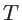the temperature,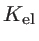andthe electrical and lattice contributions to the thermal conductivity, respectively . The numerator of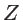is called power factor. The figure of merit determines the efficiency of a thermoelectric material (device) and can be improved by increasing the power factor and decreasing the thermal conductivity. Hence, thermoelectric materials must simultaneously have a high Seebeck coefficient, a high electrical conductivity, and a low thermal conductivity. For ballistic channels, one needs to employ conductances instead of conductivities in the Eq. 2.1. For channels in which diffusive transport prevails, however, the actual conductivity values are employed.

In this chapter, the methodology used for calculating the thermoelectric coefficients is discussed. Two steps are needed in the simulation procedure: i) material properties, a step including the calculation of electronic and phononic bandstructures and ii) transport properties, using formalisms such as the Landauer approach and Boltzmann transport theory.

317#318Next: 2.1 Graphene Up: Dissertation Hossein Karamitaheri Previous: 1.5 Graphene- and Silicon-based Nanostructures   Contents
H. Karamitaheri: Thermal and Thermoelectric Properties of Nanostructures